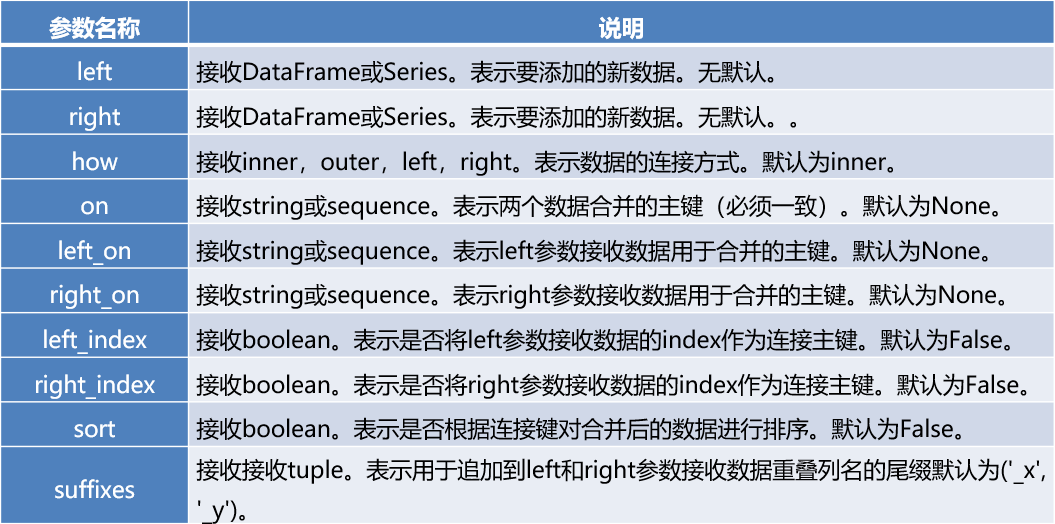# Python学习：Pandas库

pandas包含数据结构和数据处理工具的设计使得在Python中进行数据清洗和分析非常快捷。pandas经常是和NumPy,Scipy以及数据可视化工具matplotlib一起使用的。pandas支持大部分NumPy语言风格的数组计算，但最大的不同在于pandas是用于处理表格型或异质型数据的。而NumPy更适合处理同质型的数值类数组数据。

• import pandas as pd
• import numpy as np

connection_object)：从SQL表/库导入数据
• pd.DataFrame(dict)：从字典对象导入数据，Key是列名，Value是数据

• sep：用于分隔每行字段的字符序列或是正则表达式
• na_values：需要用NA替换的值序列
• skiprows：从文件开头处起，需要跳过的行数或行号列表
• nrows：从文件开头处读入的行数
• chunksize：用于迭代的块大小
• index_col：用作结果中行索引的列号或列名，可以是一个单一的名称/数字,也可以是一个分层索引
• names：结果的列名列表

• df.to_csv(filename)：导出数据到CSV文件
• df.to_excel(filename)：导出数据到Excel
• df.to_sql(table_name, connection_object)：导出数据到SQL表
• df.to_json(filename)：以Json格式导出数据到文本文件

• obj=pd.Series([4,7,-5],index=[‘a’,‘b’,‘c’])
• obj=pd.DataFrame(data,columns=[‘year’],index=[‘one’])

• df.tail(n)：查看DataFrame对象的最后n行
• df.info()：查看索引、数据类型和内存信息
• df.shape：查看行数和列数
• df.describe()：查看数值型列的汇总统计
• df.dtypes：每一列数据的格式
• df.isnull()：查看某一列空值
• df.columns ：查看列名称
• df.values：查看数据表的值
• df[‘B’].unique()：查看某一列的唯一值
• s.value_counts()：查看Series对象的唯一值和计数
• df.apply(pd.value_counts).fillna(0)：查看DataFrame对象中每一列的唯一值和计数,并对NA填充0

• df[col]：根据列名，并以Series的形式返回列
• df[[col1, col2]]：以DataFrame形式返回多列
• df[n:m]：以DataFrame形式返回n到m-1行
• df.loc[val]：根据标签选择单行或多行
• df.loc[:,val]：根据标签选择单列或多列
• df.loc[val1,val2]：同时选择行和列的一部分
• df.iloc[where]：根据整数位置选择单行或多行
• df.iloc[:,where]：根据整数位置选择单列或多列
• df.iloc[where_i,where_j]：根据整数位置选择行和列

*loc切片是首尾闭区间；iloc切片是首闭尾开

• df.columns = [‘a’,‘b’,‘c’]：重命名列名
• pd.isnull()：检查DataFrame对象中的空值
• pd.notnull()：检查DataFrame对象中的非空值
• df.dropna()：删除所有包含空值的行
• df.dropna(axis=1)：删除所有包含空值的列
• df.dropna(axis=1,thresh=n)：删除所有小于n个非空值的行
• df.fillna(x)：用x替换DataFrame对象中所有的空值
• s.astype(float)：将Series中的数据类型更改为float类型
• s.replace(1,‘one’)：用‘one’代替所有等于1的值
• s.replace([1,3],[‘one’,‘three’])：用’one’代替1，用’three’代替3
• df.rename(columns=lambda x: x + 1)：批量更改列名
• df.rename(columns={‘old_name’: ‘new_ name’})：选择性更改列名
• df.set_index(‘column_one’)：更改索引列
• df.rename(index=lambda x: x + 1)：批量重命名索引
• df.drop_duplicates() ：删除后出现的重复值
• df.drop_duplicates(keep=‘last’)：删除先出现的重复值
• df.drop_duplicates([‘k1’]) ：基于k1列删除重复值
• df[‘city’]=df[‘city’].map(str.strip)
• pd.cut(data,4) ：分箱

• df[df[col] > 0.5]：选择col列的值大于0.5的行
• df.sort_values(col1)：按照列col1排序数据，默认升序排列
• df.sort_values(col2, ascending=False)：按照列col1降序排列数据
• df.sort_values([col1,col2],ascending=[True,False])：先按列col1升序排列，后按col2降序排列数据
• df.groupby(col)：返回一个按列col进行分组的Groupby对象
• df.groupby([col1,col2])：返回一个按多列进行分组的Groupby对象
• df.groupby(‘city’)[‘id’].count() ：按城市对id字段进行计数
• df.groupby(‘city’)[‘price’].agg([np.sum,np.mean])：对city字段进行汇总，并分别计算prince的合计和均值
• df.pivot_table(index=col1,values=[col2,col3], aggfunc=max)：创建一个按列col1进行分组，并计算col2和col3的最大值的数据透视表
• df.groupby(col1).agg(np.mean)：返回按列col1分组的所有列的均值
• data.apply(np.mean)：对DataFrame中的每一列应用函数np.mean
• data.apply(np.max,axis=1)：对DataFrame中的每一行应用函数np.max

• df1.append(df2)：将df2中的行添加到df1的尾部
• pd.concat([df1, df2],axis=1)：将df2中的列添加到df1的尾部
• df1.join(df2,on=col1,how=‘inner’)：对df1的列和df2的列执行SQL形式的join
• pd.merge(df1,df2,on=‘key’) : 按指定连接健合并

*pd.concat():join接收inner或outer。表示其他轴向上的索引是按交集（inner）还是并集（outer）进行合并。默认为outer。当axis=1的时候，concat做行对齐，然后将不同列名称的两张或多张表合并。当两个表索引不完全一样时，可以使用join参数选择是内连接还是外连接。在内连接的情况下，仅仅返回索引重叠部分。在外连接的情况下，则显示索引的并集部分数据，不足的地方则使用空值填补。axis=0时同理

*df.append():append方法也可以用于纵向合并两张表。但是append方法实现纵向表堆叠有一个前提条件，那就是两张表的列名需要完全一致

*pd.merge()常用参数：• df.describe()：查看数据特征的汇总统计
• df.mean()：返回所有列的均值
• df.corr()：返回列与列之间的相关系数
• df.count()：返回每一列中的非空值的个数
• df.max()：返回每一列的最大值
• df.min()：返回每一列的最小值
• df.idxmin()：返回最小值所在的索引标签
• df.argmin()：返回最小值所在的索引位置
• df.median()：返回每一列的中位数
• df.std()：返回每一列的标准差

*传入axis=’columns’或者axis=1，则会对一行上各列值进行操作。除非整个切片都是NA，否则NA值是自动被排除的，可以通过禁用skipna来实现不排除NA值：skipna=False

04-2569
12-22393
12-08106
02-085326
05-121035
02-091万+
10-06365
11-273997
11-201250
02-052797
07-19368
12-231万+
04-242577
12-201154
04-029万+
06-102183
10-241396
09-12595
10-097380
09-181132

### “相关推荐”对你有帮助么？

•非常没帮助
•没帮助
•一般
•有帮助
•非常有帮助被折叠的  条评论 为什么被折叠?到【灌水乐园】发言¥2 ¥4 ¥6 ¥10 ¥20余额支付 (余额：-- )扫码支付获取中扫码支付点击重新获取扫码支付1.余额是钱包充值的虚拟货币，按照1:1的比例进行支付金额的抵扣。
2.余额无法直接购买下载，可以购买VIP、C币套餐、付费专栏及课程。余额充值